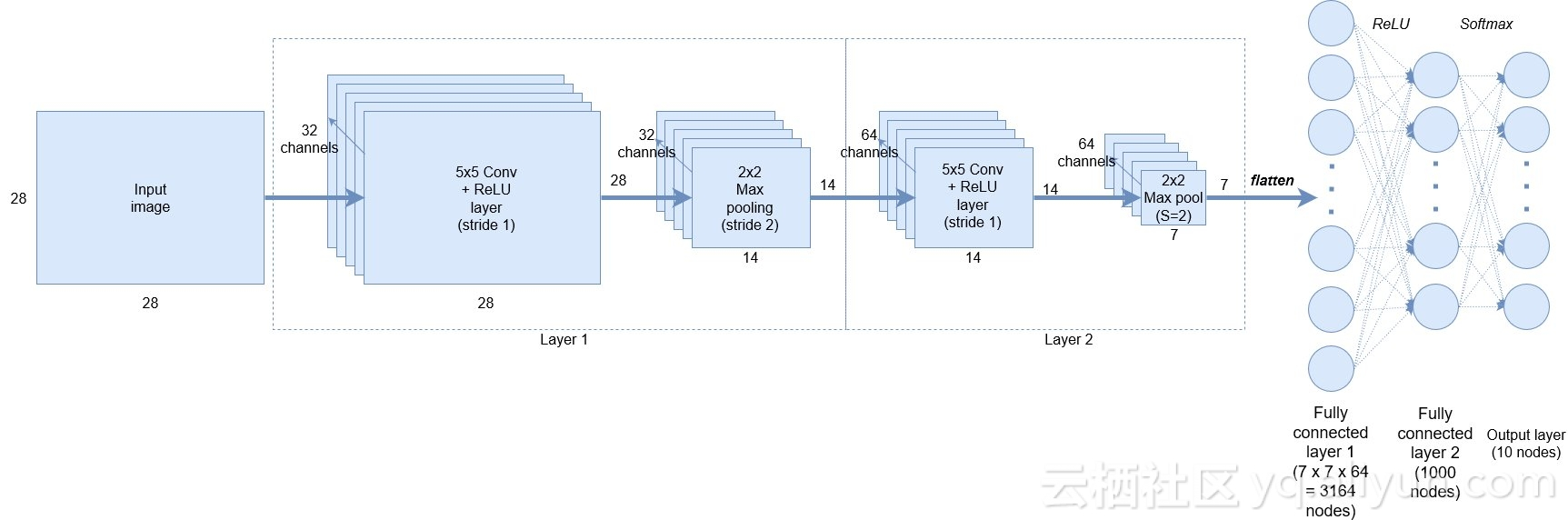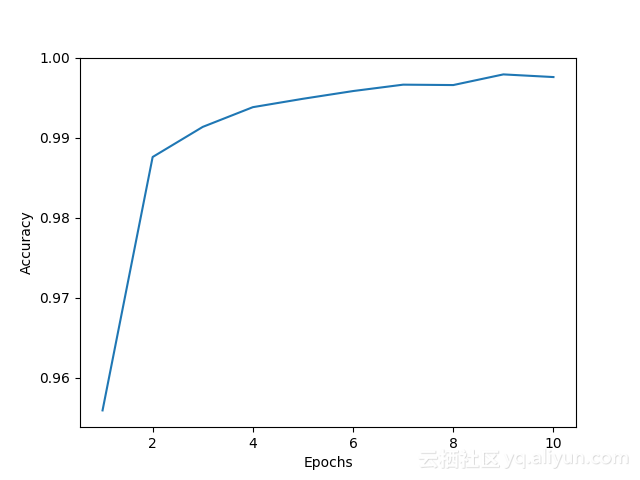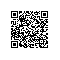# 用“Keras”11行代码构建CNNKeras教程将向你展示如何使用MNIST数据集构建CNN并实现> 99％的准确性。它与我之前的卷积神经网络教程中构建的结构完全相同 ，下图显示了网络的架构：## Keras教程中的主要代码讲解：

model = Sequential()
activation='relu',
input_shape=input_shape))


Model  =  Sequential()

Keras中的模型可以有两种——序贯和通过API函数。对于构建的大多数深度学习网络，序贯模型是最常用的。它允许你从输入到输出整个过程都能轻松地堆叠网络层（甚至循环层）。而API函数可以帮助你构建更复杂的网络体系结构，本教程将不介绍它。

model.add(Conv2D(32, kernel_size=(5, 5), strides=(1, 1),
activation='relu',
input_shape=input_shape))


model.add(MaxPooling2D(pool_size=(2, 2), strides=(2, 2)))

model.add(Conv2D(64, (5, 5), activation='relu'))
model.add(MaxPooling2D(pool_size=(2, 2)))

model.add(Flatten())


## 训练和评估卷积神经网络

model.compile(loss=keras.losses.categorical_crossentropy,
optimizer=keras.optimizers.SGD(lr=0.01),
metrics=['accuracy'])


model.fit(x_train, y_train,
batch_size=batch_size,
epochs=epochs,
verbose=1,
validation_data=(x_test, y_test),
callbacks=[history])


3328/60000 [>.............................] - ETA: 87s - loss: 0.2180 - acc: 0.9336
3456/60000 [>.............................] - ETA: 87s - loss: 0.2158 - acc: 0.9349
3584/60000 [>.............................] - ETA: 87s - loss: 0.2145 - acc: 0.9350
3712/60000 [>.............................] - ETA: 86s - loss: 0.2150 - acc: 0.9348

score = model.evaluate(x_test, y_test, verbose=0)
print('Test loss:', score)
print('Test accuracy:', score)Kersa让事情很更加简单了，你不觉得吗？我希望这个Keras教程已经展示了它如何成为深度学习解决方案的有用框架。

## 在Keras中记录网络性能

Keras有一个实用的程序，名为回调，可用于跟踪训练期间的各种变量。你还可以使用它来创建检查点，将模型在训练的不同阶段进行保存，以帮助你避免工作结果丢失。整个结果会被传递到.fit（）函数，如上所述。我会向你显示一个相当简单的用例，其中记录了准确性。

class AccuracyHistory(keras.callbacks.Callback):
def   on_train_begin(self, logs={}):
self.acc = []
def  on_epoch_end(self, batch, logs={}):
self.acc.append(logs.get('acc'))

history = AccuracyHistory()

plt.plot(range(1,11), history.acc)
plt.xlabel('Epochs')
plt.ylabel('Accuracy')
plt.show()使用钉钉扫一扫加入圈子
+ 订阅